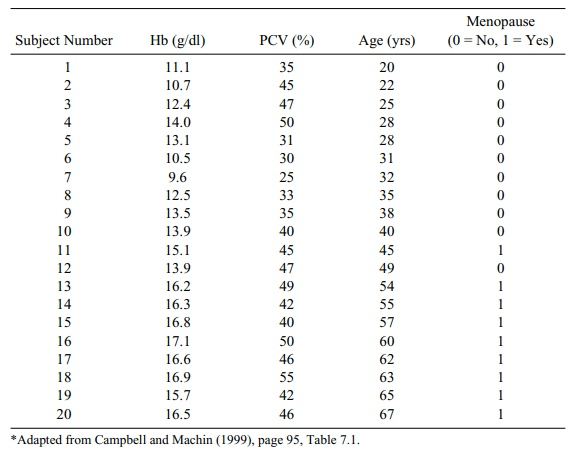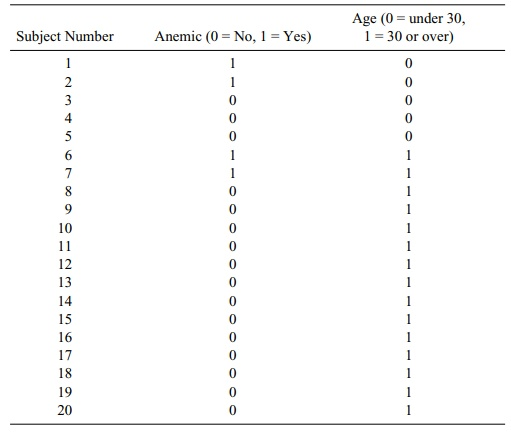# Logistic Regression

| Home | | Advanced Mathematics |

## Chapter: Biostatistics for the Health Sciences: Correlation, Linear Regression, and Logistic Regression

Logistic regression is a method for predicting binary outcomes on the basis of one or more predictor variables (covariates).

LOGISTIC REGRESSION

Logistic regression is a method for predicting binary outcomes on the basis of one or more predictor variables (covariates). The goal of logistic regression is the same as the goal of ordinary multiple linear regression; we attempt to construct a model to best describe the relationship between a response variable and one or more inde-pendent explanatory variables (also called predictor variables or covariates). Just as in ordinary linear regression, the form of the model is linear with respect to the re-gression parameters (coefficients). The only difference that distinguishes logistic regression from ordinary linear regression is the fact that in logistic regression the response variable is binary (also called dichotomous), whereas in ordinary linear re-gression it is continuous.

A dichotomous response variable requires that we use a methodology that is very different from the one employed in ordinary linear regression. Hosmer and Lemeshow (2000) wrote a text devoted entirely to the methodology and many im-portant applications of handling dichotomous response variables in logistic regres-sion equations. The same authors cover the difficult but very important practical problem of model building where a “best” subset of possible predictor variables is to be selected based on data. For more information, consult Hosmer and Lemeshow (2000).

In this section, we will present a simple example along with its solution. Given that the response variable Y is binary, we will describe it as a random variable that takes on either the value 0 or the value 1. In a simple logistic regression equation with one predictor variable, X, we denote by π(x) the probability that the response variable Y equals 1 given that X = x. Since Y takes on only the values 0 and 1, this probability π(x) also is equal to E(Y|X = x) since E(Y|X = x) = 0 P(Y = 0|X = x) + 1 P(Y = 1|X = x) = P(Y = 1|X = x) = π(x).

Just as in simple linear regression, the regression function for logistic regression is the expected value of the response variable, given that the predictor variable X = x. As in ordinary linear regression we express this function by a linear relationship of the coefficients applied to the predictor variables. The linear relationship is spec-ified after making a transformation. If X is continuous, in general X can take on all values in the range (–`, +`). However, Y is a dichotomy and can be only 0 or 1. The expectation for Y, given X = x, is that π(x) can belong only to [0, 1]. A linear combination such as α + βx can be in (–`, +`) for continuous variables. So we con-sider the logit transformation, namely g(x) = ln[π(x)/(1 – π(x)]. Here the transfor-mation w(x) = [π(x)/(1 – π(x)] can take a value from [0, 1] to [0, +`) and ln (the logarithm to the base e) takes w(x) to (–`, +`). So this logit transformation puts g(x) in the same interval as α + βx for arbitrary values of α and β.

The logistic regression model is then expressed simply as g(x) = α + βx where g is the logit transform of π. Another way to express this relationship is on a probability scale by reversing (taking the inverse) the transformations, which gives π(x) = exp(α + βx)/[1 + exp(α + βx)], where exp is the exponential function. This is because the exponential is the inverse of the function ln. That means that exp(ln(x)) = x. So exp[g(x)] = exp(α + βx) = exp{ln[π(x)/(1 – π(x)]} = π(x)/1 – π(x). We then solve exp(α + βx) = π(x)/1 – π(x) for π(x) and get π(x) = exp(α + βx)[1 – π(x)] = exp(α + βx) – exp(α + βx)π(x). After moving exp(α + βx) π(x) to the other side of the equation, we have π(x) + exp(α + βx)π(x) = exp(α + βx) or π(x)[1 + exp(α + βx)] = exp(α + βx). Dividing both sides of the equation by 1 + exp(α + βx) at last gives us π(x) = exp(α + βx)/[1 + exp(α + βx)].

The aim of logistic regression is to find estimates of the parameters α and β that best fit an available set of data. In ordinary linear regression, we based this estima-tion on the assumption that the conditional distribution of Y given X = x was nor-mal. Here we cannot make that assumption, as Y is binary and the error term for Y given X = x takes on one of only two values, –π(x) when Y = 0 and 1 – π(x) when Y = 1 with probabilities 1 – π(x) and π(x), respectively. The error term has mean zero and variance [1 – π(x)]π(x). Thus, the error term is just a Bernoulli random variable shifted down by π(x).

The least squares solution was used in ordinary linear regression under the usual assumption of constant variance. In the case of ordinary linear regression, we were told that the maximum likelihood solution was the same as the least squares solu-tion [Draper and Smith (1998), page 137, and discussed in Sections 12.8 and 12.10 above]. Because the distribution of error terms is much different for logistic regres-sion than for ordinary linear regression, the least squares solution no longer applies; we can follow the principle of maximizing the likelihood to obtain a sensible solu-tion. Given a set of data (yi, xi) where i = 1, 2, . . . , n and the yi are the observed re-sponses and can have a value of either 0 or 1, and the xi are the corresponding co-variate values, we define the likelihood function as follows:

L(x1, y1, x2, y2, . . . , xn, yn) = π(x1)y1[1 – π(x1)](1–y1) π(x2)y2[1 – π (x2)](1–y2) π(x3)y3[1 – π(xn)](1–y3), . . . , π(xn)yn[1 – π(xn)](1–yn)                                  (12.1)

This formula specifies that if yi = 0, then the probability that yi = 0 is 1 – π(xi); whereas, if yi = 1, then the probability of yi = 1 is π(xi). The expression π(xi)yi[1 – π(xi)](1–yi) provides a compact way of expressing the probabilities for yi = 0 or yi = 1 for each i regardless of the value of y. These terms shown on the right side of the equal sign of Equation 12.8 are multiplied in the likelihood equation because the observed data are assumed to be independent. To find the maximum values of the likelihood we solve for α and β by simply computing their partial derivatives and setting them equal to zero. This computation leads to the likelihood equations Σ[yiπ(xi)] = 0 and Σxi[yi π(xi)] = 0 which we solve simultaneously for α and β. Recall that in the likelihood equations π(xi) = exp(α + βxi)/[1 + exp(α + βxi)], so the parameters α and β enter the likelihood equations through the terms with π(xi).

Generalized linear models are linear models for a function g(x). The function g is called the link function. Logistic regression is a special case where the logit func-tion is the link function. See Hosmer and Lemeshow (2000) and McCullagh and Nelder (1989) for more details.

Iterative numerical algorithms for generalized linear models are required to solve maximum likelihood equations. Software packages for generalized linear models provide solutions to the complex equations required for logistic regression analysis. These programs allow you to do the same things we did with ordinary sim-ple linear regression—namely, to test hypotheses about the coefficients (e.g., whether or not they are zero) or to construct confidence intervals for the coeffi-cients. In many applications, we are interested only in the predicted values π(x) for given values of x.

Table 12.10 reproduces data from Campbell and Machin (1999) regarding he-moglobin levels among menopausal and nonmenopausal women. We use these data in order to illustrate logistic regression analysis.

Campbell and Machin used the data presented in Table 12.10 to construct a lo-gistic regression model, which addressed the risk of anemia among women who were younger than 30. Female patients who had hemoglobin levels below 12 g/dl were categorized as anemic. The present authors (Chernick and Friis) dichotomized the subjects into anemic and nonanemic in order to examine the relationship of age (under and over 30 years of age) to anemia. (Refer to Table 12.11.)

We note from the data that two out of the five women under 30 years of age were anemic, while only two out of 15 women over 30 were anemic. None of the women who were experiencing menopause was anemic. Due to blood and hemoglobin loss during menstruation, younger, nonmenopausal women (in comparison to menopausal women) were hypothesized to be at higher risk for anemia.

In fitting a logistic regression model for anemia as a function of the di-chotomized age variable, Campbell and Machin found that the estimate of the re-gression parameter β was 1.4663 with a standard error of 1.1875. The Wald test, analogous to the t test for the significance of a regression coefficient in ordinary lin-ear regression, is used in logistic regression. It also evaluates whether the logistic regression coefficient is significantly different from 0. The value of the Wald statis-tic was 1.5246 for these data (p = 0.2169, n.s.).

TABLE 12.10. Hemoglobin Level (Hb), Packed Cell Volume (PCV), Age, and Menopausal Status for 20 Women*TABLE 12.11. Women Reclassified by Age Group and Anemia (Using Data from Table 12.10)With such a small sample size (n = 20) and the dichotomization used, one cannot find a statistically significant relationship between younger age and anemia. We can also examine the exponential of the parameter estimate. This exponential is the estimated odds ratio (OR), defined elsewhere in this book. The OR turns out to be 4.33, but the confidence interval is very wide and contains 0.

Had we performed the logistic regression using the actual age instead of the dichotomous values, we would have obtained a coefficient of –0.2077 with a standard error of 0.1223 for the regression parameter, indicating a decreasing risk of anemia with increasing age. In this case, the Wald statistic is 2.8837 (p = 0.0895), indicating that the downward trend is statistically significant at the 10% level even for this relatively small sample.